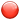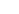## Training Snap Shot: Learning Formulas

### WHAT ARE FORMULAS?

•The start of a formula.=SUM – The total value of the cell range.=SUM(A1:A2, - This is the process of adding multiple cell ranges to the formula.=SUMIF – Adds the cells specified by a given condition or criteria.=COUNT – Counts the number of cells in a range that contain numbers.=COUNTA – Counts the number of cells in a range that are not empty.=COUNTBLANK – Counts the number of empty cells in a specified range of cells.=COUNTIF – Counts number of cells within a range that meet the given condition.

•EXCEL HELP FILE
A reference identifies a cell or a range of cells on a worksheet, and tells Excel where to look for the values or data you want to use in a formula. You can use references to use data contained in different parts of a worksheet in one formula or use the value from one cell in several formulas.
You can also refer to cells on other sheets in the same workbook, and to other workbooks. References to cells in other workbooks are called links or external references.

By default, Excel uses the A1 reference style, which refers to columns with letters (A through XFD, for a total of 16,384 columns) and refers to rows with numbers (1 through 1,048,576). These letters and numbers are called row and column headings. To refer to a cell, enter the column letter followed by the row number. For example, B2 refers to the cell at the intersection of column B and row 2.

A relative cell reference in a formula, such as A1, is based on the relative position of the cell that contains the formula and the cell the reference refers to. If the position of the cell that contains the formula changes, the reference is changed. If you copy or fill the formula across rows or down columns, the reference automatically adjusts. By default, new formulas use relative references. For example, if you copy or fill a relative reference in cell B2 to cell B3, it automatically adjusts from =A1 to =A2.